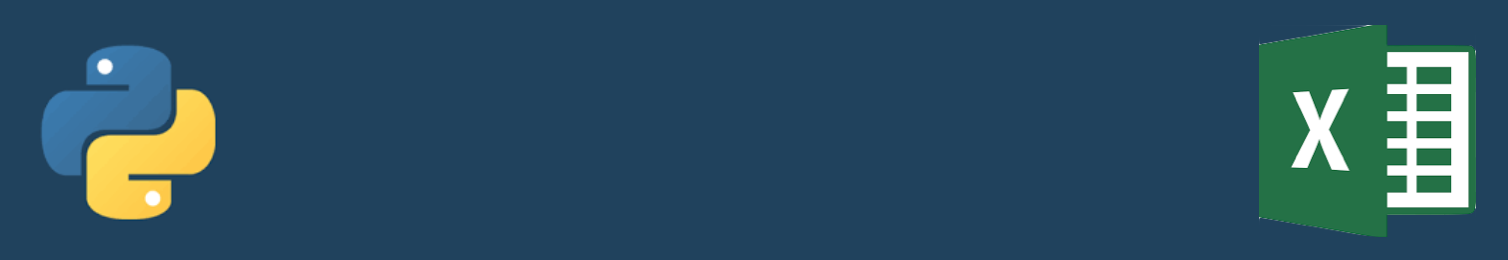# How to control Excel with Python

There are several packages which allow manipulation of excel spreadsheets in python.

## xlrd, xlwt

Pronounced "Excel read" and "Excel write" these two packages enable simple spreadsheet manipulation. You can find a tutorial here.

## Python Extension for Windows

"Python Extension Package for Windows", "Python Windows Extension", "pywin32" are all referencing the same thing. It is a package written which enables direct control of various aspects of the Windows operating system, including control of Excel. Christoph Gohlke has helpfully created an easy installer. Once you've installed it, here is a tutorial for using it to manipulate Excel spreadsheets. The cool thing about it is that it enables you to see what it is doing; Excel actually opens and performs the commands you want.

Here is a bit of code which opens a spreadsheet, manipulates it, and saves it.

import win32com.client
excel = win32com.client.Dispatch("Excel.Application")
excel.Visible = True
workbook = excel.Workbooks.Open("D:\Desktop\simple_example.xlsx")
sheet = workbook.Worksheets(1) #workbook.Sheets('Sheet1').Select();
sheet = xlApp.ActiveSheet
sheet.Cells(1,1).Value="Hello"
workbook.Save()
workbook.Close()
excel.Quit()
sheet = None
book = None
excel.Quit()
excel = None


## pandas

Pandas allows you to read an excel table and converts it into the useful Pandas dataframe. The read function is

pandas.io.excel.read_excel('workbook.xlsx')


## openpyxl

OpenPyXL is another excel opening package. Here is a guide for simple commands. To read a file:

from openpyxl import load_workbook